MathExamle.com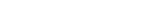#### Welcome to the site MathExample.com

This educational resource is intended for you to acquire skills in practical mathematics, as well as for the development of mathematical abilities in you and your children.

" The numbers are a catalyst that can help turn raving mad men into polite humans"

~Philip J. Davis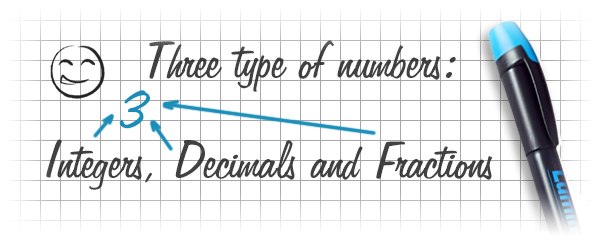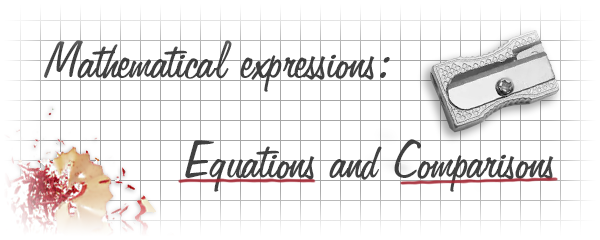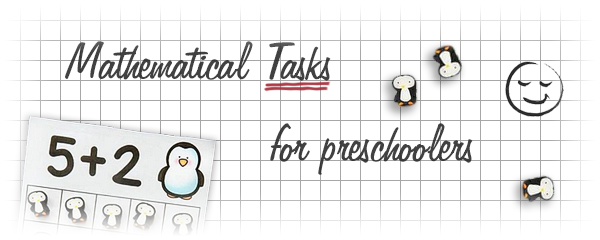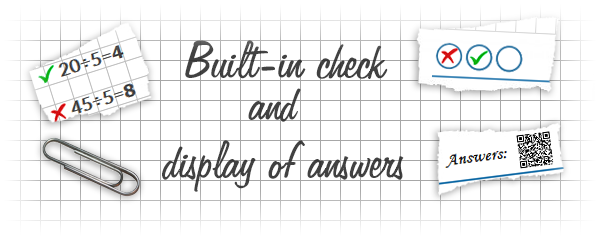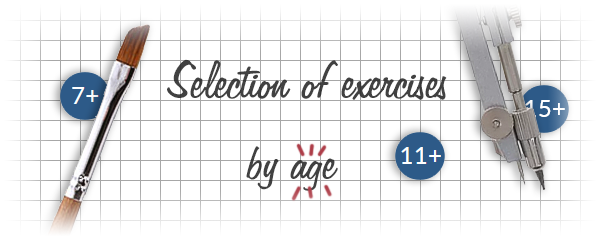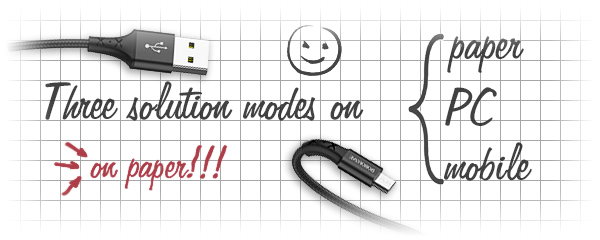Website Goals:

• increased knowledge in mathematics
• gaining practical experience in solving mathematical tasks
• increasing the speed and quality of logical and mathematical thinking
• development of memory, mindfulness and concentration

Using this resource you can:

• gain basic knowledge of addition, subtraction, multiplication and division
• learn the multiplication table with practical exercises
• learn operations with integer, decimal and fractional numbers
• master the methods of solving equations
• get hands-on spelling

The site provides the following features:

• creation of tasks of any complexity
• printing worksheets of any size
• solving and fixing results online
• built-in check and display of answers by link or QR code
• two modes on a computer and on a mobile device

The ability to think rationally needs to be developed and consolidated, it is easier to do with us!

8+

Create worksheets of mathematical examples for addition and subtraction by a column method. The answers and numbers of a example are integer and positive. Total 3 variants and 3 levels of complexity.

Creates examples for addition or subtraction of integers by a column method

3 Variants 3 Levels
 – 90 60
 – 79 31
 – 99 89
 – 92 14
Variant: 2 Level: Easy
 Sheet
10+

Creates worksheets with English infinitives where some words are missing. Missing verbs must be completed. Only 3 options and 3 difficulty levels.

Creates worksheets with English infinitives where some words are missing, missing verbs must be completed

3 Variants 3 Levels
 undersell undersold [undersell] outthink outthought [outthink]
Variant: 1 Level: Easy
 vocabulary Sheet
6+

Step-by-step addition task for each day. Develops the ability to solve examples with the addition operation.

 9+3= 1+3= 3+9=
Attempts: 2 Level: Light
11+

Create worksheets of mathematical examples for multiplication by a column method. The answers and numbers of a example are integer and positive. Total 1 variant and 5 levels of complexity.

Creates examples with a multiplication operation of integers by a column method

1 Variant 5 Levels
 8118×869= 85000×103= 2250×908= 11125×800=
Variant: 1 Level: Difficult
 Sheet
14+

Create worksheets of quadratic equations. The numbers of a equation are integers. The variables can be negative. Total 3 variants and 3 levels of complexity.

Creates quadtatic equations, the answers are integers and can be negative

3 Variants 3 Levels
 10x2=0 10x2=90 4x2=256 5x2=0 2x2=0 5x2=80
Variant: 1 Level: Easy
 Sheet

## Visitors MathExample.com

 If you have any questions or requests to improve this site, please contact us. If errors occur on the site, please also write to our developers.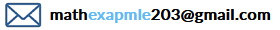## Share the link

If you have the opportunity, please let your friends and acquaintances know about us, we will be very grateful to you.